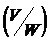# MATHEMATICS MODEL PAPER FIFTH SEMESTER PAPER 6 – LINEAR ALGEBRA COMMON FOR B.A & B.Sc (w.e.f. 2015-16 admitted batch)

 MATHEMATICS MODEL PAPER FIFTH SEMESTER  PAPER 6 – LINEAR ALGEBRA COMMON FOR B.A & B.Sc  (w.e.f. 2015-16 admitted batch) SECTION – A I. Answer any five questions. Each question carries five marks. 5 x 5 = 25 M 1. Let p, q, r be the fixed elements of a field F. Show that the set W of all triads (x, y, z) of elements of F, such that px + qy + rz = 0 is a vector subspace of V3(F). 2. Express the vector a = (1, –2, 5) as a linear combination of the vectors e1 = (1, 1, 1), e2 = (1, 2, 3) and e3 = (2, –1, 1) 3. If a, b, g are L.I vectors of the vector space V(R) then show that a + b, b + g, g + a are also L. I vectors 4 Describe explicitly the linear transformation T : R2 ®  R2 such that T (1, 2) = 3,0) and T(2,1) = (1, 2) 5. Let U(F) and V(F0 be two vector space and T : U(F) ® V(F) be a linear transformation. Prove that the range set R(T) is a substance of V(F). 6. Solve the system 2x – 3y + z = 0, x + 2y – 3z = 0, 4x – y – 2z = 0 7. State and prove Schwarz inequality. 8. is an orthogonal set of the inner product space R3® SECTION – B II. Answer the all five questions. Each carries ten marks 5 x 10 = 50 M 9. a) Prove that the subspace W to be a subspace of V(F) Û aa + bb Î W "a, b Î F and a, bÎ W. (OR) b) Prove that the four vectors a = (1, 0, 0), b = (0, 1, 0), g = (0, 0, 1), d =(1, 1, 1) in V3(C) form a linear dependent set, but any three of them are linear independent. 10. a) Let W be a subspace of finite dimensional vector space V(F), then prove that dim= dim (V) – dim (W). (OR) b) Let W1 and W2 be two subspaces of a finite dimensional vector space V(F). Then prove that dim (W1 + W2) = dim (W1) + dim (W2) – dim (W1 Ç W2) 11. a) State and prove Rank-Nullity theorem. (OR) b) Define a linear transformation. Show that the mapping T : R3 ® R2 is defined by T (x, y, z) = (x – y, x –z) is a linear transformation 12. a) State and prove Cayley –Hamilton theorem. (OR) b) Find the characteristic roots and the corresponding characteristic vectors of the matrix 13. a) State and prove Bessel’s inequality. (OR) b) Applying Gram-Schmidt orthogonalization process to obtain an orthonormal basis of R3(R) from the basis

THE END

#### Related

Model Papers 5931364344555837413